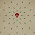## Wednesday, March 3, 2010

### Arrange multiple ggplot2 plots in the same image window

In a previous tutorial I showed you how to create plots faceted by the level of a third variable using ggplot2. A commenter asked about using faceted plots and viewports and reminded me of this function I found in the ggplot2 Google group. The arrange function below is similar to using par(mfrow=c(r,c)) in base graphics to put more than one plot in the same image window.

The basic idea is that you assign ggplot2 plots to an object, and then use the arrange function to display two or more. Here's an example. First copy and paste the code above (or put in your Rprofile). Next install and/or load ggplot2 as described in a previous ggplot2 tutorial.

```# Load the diamonds dataset
data(diamonds)

# Create a histogram, assign to "plot1"
plot1 <- qplot(price,data=diamonds,binwidth=1000)

# Create a scatterplot
plot2 <- qplot(carat,price,data=diamonds)

# Arrange and display the plots into a 2x1 grid
arrange_ggplot2(plot1,plot2,ncol=1)
```
And here's what you should get:

...

1.wow that was fast :)

2.Also, check out imagemagick. It's a cross platform opensource image manipulation tool. It's really handy for stitching multiple images together. But the montage binary in your path, then call:

montage image1.png image2.png -geometry -0-0 -tile 1x composite.png

to stitch image1 and image2 together in a single column. Ideally all your images will be the same size. You can do this with lots of images using wildcards.

3.Great tip! I've been struggling with this aspect of ggplot2 for a while.

4.Great!! I was looking for something like this just today!

5.I started learning ggplot2 a couple weeks ago, so maybe I'm missing something. After I load the library(ggplot2), arrange() isn't showing up as a function. I tried loading library(ggplot), to see if it was in there, but still no luck.

Any idea? ... I've been looking for this solution, but the no-arrange() is getting in the way.

6.Dan, the arrange function is not included with ggplot2. You have to copy and paste the code in the first box above to get it to work.

7.woohoo! works great! thanks

8.Great bit of code... But.. is there a way to output to a file?

I've tried:-
tmp<-arrange(mh3,mh4,mh1,mh2,ncol=1)
ggsave(file="all.png",tmp)

9.Jennie,

I haven't had success with this either. Just use the GUI to save the image as PNG or PDF. I'll post a solution if I find one.

Stephen

10.to save plots to file

pdf("your_file.pdf",width = 11, height = 8)
arrange(plot1,plot2,plot3,plot4,ncol=2)
dev.off()

11.Hi,
I get the following error when I do this.
Error: attempt to apply non-function

12.Hey guys, can you slap a license on your code? (BSD would be highly appreciated)

13.This comment has been removed by the author.

14.note that this function is included in the gridExtra package, with the name grid.arrange() and a few more options.

15.Great trick, thanks. How to go about having two plots share the same x-axis?

16.Thank you Stephen Turner. It has been great use your code. Thanks.

17.how do you control the space between the plots when using "arrange function"?

18.i put your code from above in R and run it and than told him to arrange my plots, but i always get the error message

couldn't find function "grid.newpage"

any idea what i could do differently?

1.Apologies - I updated the code (and renamed the function to arrange_ggplot2). The problem is newer versions of ggplot2 don't automatically load the grid package, so you have to load it manually. I put this in as the first line of code. Give it a shot now.

19.Thanks Steven! My google searches seem to end up on your blog fairly often with helpful results. I also found this package (http://code.google.com/p/gridextra/) which may be useful as well.

1.Thanks for sharing. I modified this code above years ago from something I found in a mailing list or forum. I think eventually this functionality became part of the gridextra package.

20.Thanks Steven for the code. It´s has been very useful !!
One question. I have a plot of 5x3 and I would like to reduce the blank space between plots. Is it possible?
Thanks
Juan Carlos

1.I am wondering the same thing here. I see that you cannot simply change

theme(plot.margin = ...)

is the margin something that is a constant when using this function or is it an function of the plot size? Also how can we reduce this and increase size of plots?

21.Perfect - Thank you!

Note: Only a member of this blog may post a comment.